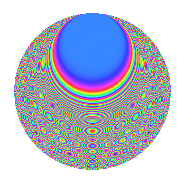# Properties

 Label 864.2.yLevel 864 Weight 2 Character orbit y Rep. character $$\chi_{864}(97,\cdot)$$ Character field $$\Q(\zeta_{9})$$ Dimension 216 Newform subspaces 4 Sturm bound 288 Trace bound 11

# Related objects

## Defining parameters

 Level: $$N$$ = $$864 = 2^{5} \cdot 3^{3}$$ Weight: $$k$$ = $$2$$ Character orbit: $$[\chi]$$ = 864.y (of order $$9$$ and degree $$6$$) Character conductor: $$\operatorname{cond}(\chi)$$ = $$27$$ Character field: $$\Q(\zeta_{9})$$ Newform subspaces: $$4$$ Sturm bound: $$288$$ Trace bound: $$11$$ Distinguishing $$T_p$$: $$5$$, $$7$$

## Dimensions

The following table gives the dimensions of various subspaces of $$M_{2}(864, [\chi])$$.

Total New Old
Modular forms 912 216 696
Cusp forms 816 216 600
Eisenstein series 96 0 96

## Trace form

 $$216q + O(q^{10})$$ $$216q + 24q^{29} - 36q^{33} - 36q^{41} + 24q^{45} + 12q^{57} + 48q^{65} + 48q^{69} + 48q^{77} + 48q^{81} - 12q^{89} + 144q^{93} + 36q^{97} + O(q^{100})$$

## Decomposition of $$S_{2}^{\mathrm{new}}(864, [\chi])$$ into newform subspaces

Label Dim. $$A$$ Field CM Traces $q$-expansion
$$a_2$$ $$a_3$$ $$a_5$$ $$a_7$$
864.2.y.a $$48$$ $$6.899$$ None $$0$$ $$0$$ $$0$$ $$0$$
864.2.y.b $$54$$ $$6.899$$ None $$0$$ $$0$$ $$0$$ $$0$$
864.2.y.c $$54$$ $$6.899$$ None $$0$$ $$0$$ $$0$$ $$0$$
864.2.y.d $$60$$ $$6.899$$ None $$0$$ $$0$$ $$0$$ $$0$$

## Decomposition of $$S_{2}^{\mathrm{old}}(864, [\chi])$$ into lower level spaces

$$S_{2}^{\mathrm{old}}(864, [\chi]) \cong$$ $$S_{2}^{\mathrm{new}}(27, [\chi])$$$$^{\oplus 6}$$$$\oplus$$$$S_{2}^{\mathrm{new}}(54, [\chi])$$$$^{\oplus 5}$$$$\oplus$$$$S_{2}^{\mathrm{new}}(108, [\chi])$$$$^{\oplus 4}$$$$\oplus$$$$S_{2}^{\mathrm{new}}(216, [\chi])$$$$^{\oplus 3}$$$$\oplus$$$$S_{2}^{\mathrm{new}}(432, [\chi])$$$$^{\oplus 2}$$

## Hecke Characteristic Polynomials

There are no characteristic polynomials of Hecke operators in the database